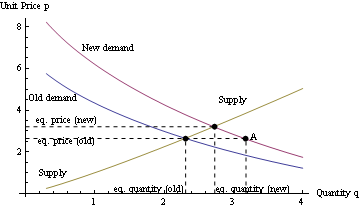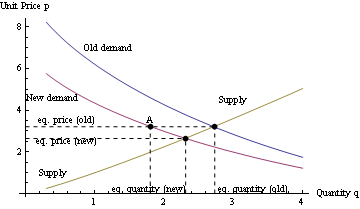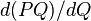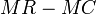# Comparative statics for demand and supply

(diff) ← Older revision | Latest revision (diff) | Newer revision → (diff)

This article discusses the comparative statics for demand curves and supply curves with particular interest on the effect of the movement of these curves on the equilibrium quantity traded and the market price.

For simplicity, we assume that all demand curves are downward-sloping, i.e., that the law of demand holds. However, for the supply curves, we consider both upward-sloping and downward-sloping supply curves. While short-run supply curves are almost always upward-sloping, long-run supply curves may be upward-sloping, flat, or downward-sloping, depending on whether the industry is an increasing cost industry, constant cost industry, or decreasing cost industry.

## Upward-sloping supply curve: Short run and competitive markets

This section builds on an understanding of market price and convergence towards market price

This is the typical case, particularly for a short-run analysis. This is because the short-run supply curve is almost always upward-sloping.

All subcases here can be handled purely by geometric visualization: we start with the canonical picture of an intersecting downward-sloping demand curve and upward-sloping supply curve. We then shift the relevant curve in the relevant direction, and see how the price and quantity coordinate of the new intersection point compare with those of the old.

Comparative change Possible causes Effect on equilibrium price Effect on equilibrium quantity traded
expansion of demand curve, i.e., the demand curve moves upward/rightward increase in market size, increase in income, increase in price of substitute goods, decrease in price of complementary goods, change in tastes and preferences, change in climate conditions, change in expectations of future prices increases increases
contraction of demand curve, i.e., the demand curve moves downward/leftward decrease in market size, decrease in income, decrease in price of substitute goods, increase in price of complementary goods, change in tastes and preferences, change in climate conditions, change in expectations of future prices decreases decreases
expansion of supply curve, i.e., the supply curve moves downward/rightward increase in number of suppliers, reduction in cost of production due to reduction in prices of factors of production decreases increases
contraction of supply curve, i.e., the supply curve moves upward/leftward decrease in number of suppliers, increase in cost of production due to increase in prices of factors of production increases decreases

### Changes in the demand curve

Further information: Convergence towards market priceWhen the demand curve expands from the blue to the purple curve, demand increases at the old equilibrium price to A, leading to a shortfall. Price and supply increase till a new equilibrium is attainedWhen the demand curve contracts from the blue to the purple curve, demand decreases at the old equilibrium price to A, leading to a surplus. Price and supply decrease till a new equilibrium is attained

We have the following basic observations:

• An outward (or rightward) shift in the demand curve leads to an increase both in the market price and in the equilibrium quantity traded. This increase is achieved by the mechanism discussed above: temporary scarcity is created, suppliers respond by increasing price and production, and buyers respond by reducing their demand from the increased value. For more on how this process works, see the convergence towards market price page.
• An inward (or leftward) shift in the demand curve leads to a decrease both in the market price and in the equilibrium quantity traded. This decrease is achieved by the mechanism discussed above: a surplus is created, suppliers try to get rid of it by reducing quantity produced as well as prices, and buyers respond by increasing their demand from the reduced value. For more on how this process works, see the convergence towards market price page.

Note that in certain situations, the demand curve may change shape in a way that is not uniformly inward or outward (for instance, this happens when there are changes in the income distribution over the collection of households being considered).

Here is how the various determinants of demand (typically) influence the demand curve:

• Income (the income effect): An increase in income typically causes the demand curve to move outward (i.e., more demand at the same price). The exception is for inferior goods.
• Prices of substitutes (the substitution effect): An increase in the price of substitutes causes the demand curve to move outward, while a decrease in the price of substitutes causes the demand curve to move inward.
• Prices of complementary goods: An increase in the price of complements typically causes the demand curve to move inward, and a decrease in the price of complements causes the demand curve to move outward.
• Expectation of future prices: For goods where it is possible to choose when to consume it (hence either hasten or delay gratification), the expectation of lower prices in the future causes an inward shift of the demand curve, and the expectation of high prices in the future causes an outward shift of the demand curve. In contrast, if current consumption is complementary to future consumption, the opposite effect may be encountered.
• Preferences.

### Changes in the supply curve

We have the following basic observations:

• An outward (or rightward) shift of the supply curve leads to a decrease in the market price and an increase in the equilibrium quantity traded.
• An inward (or leftward) shift of the supply curve leads to an increase in the market price and a decrease in the equilibrium quantity traded.

A sudden shift in the supply curve is termed a supply shock. Supply shocks for important commodities could have major effects on the economy.

## Downward-sloping supply curve: long run and competitive markets

This case is important for the analysis of long-run supply curve, i.e., for long run price trends, in a decreasing cost industry.

When both the demand curve and the supply curve are downward-sloping, it is possible for them to have more than one point of intersection. The relevant market price and equilibrium quantity traded occur at a point beyond which the supply curve becomes farther right of the demand curve.

Thus, for simplicity, we assume a situation where the supply curve is downward-sloping but is flatter (more horizontal) than the demand curve. In this case, there is a unique point of intersection of the curves and this gives the market price and equilibrium quantity traded.

Comparative change Possible causes Effect on equilibrium price Effect on equilibrium quantity traded
expansion of demand curve, i.e., the demand curve moves upward/rightward increase in market size, increase in income, increase in price of substitute goods, decrease in price of complementary goods, change in tastes and preferences, change in climate conditions, change in expectations of future prices decreases increases
contraction of demand curve, i.e., the demand curve moves downward/leftward decrease in market size, decrease in income, decrease in price of substitute goods, increase in price of complementary goods, change in tastes and preferences, change in climate conditions, change in expectations of future prices increases decreases

## Monopolistic markets in the short run

This section builds on an understanding of determination of price and quantity supplied by monopolistic firm in the short run

For a market with only one seller, there is no concept of a supply curve. Rather, the firm looks at the market demand curve and simultaneously optimizes for both price and quantity to maximize profits. When the demand curve shifts, the firm has to solve a new optimization problem.

The optimal (price, quantity) pair the firm chooses is determined by the point of crossing of the marginal cost curve of the firm (based on the firm's production technology) and the marginal revenue curve (obtained from the market demand curve as the derivative$d(PQ)/dQ$ treating$P$ as the inverse demand function of$Q$, with graph the market demand curve), with$MR - MC$ switching sign from positive to negative. Unlike the case of competitive markets, where the slopes of the demand and supply curve are clearly understood, the picture here is unclear: the marginal revenue curve may be upward-sloping, downward-sloping, or mixed, depending on how the price-elasticity of demand compares with 1 (in magnitude).

### Effect of expansion of the demand curve

Very little can be said in general about the effect of expansions of contractions of the demand curve on the price and quantity supplied, because what matters are the shape changes. The following are the two main statements that can be made:

• The firm's profit goes up under an expansion of the demand curve: For this, note that sticking to the same quantity of production and adjusting to the new price will, in and of itself, raise the firm's profit. Therefore, moving to the new optimal point would make the firm's profit go up even further.
• It is not possible for both price and quantity to decrease due to an expansion of the demand curve (see explanation in the table below).
Effect on optimal price of expansion of demand curve Effect on optimal quantity of expansion of demand curve Scenario type
increases increases The increased demand makes it desirable to serve a larger market, but not enough to lower the price: expanding the market to the point that it might necessitate lowering the price is not worthwhile because the population gained that way is not large enough to compensate for the lower producer surplus per sale. This scenario qualitatively resembles competitive markets.
increases decreases Demand increases a lot among the segment with the highest reservation price, so that the optimal choice is to raise prices to capture a larger share of the social surplus from selling to these customers, even at the cost of losing out other segments.
decreases increases There is increased demand from a share of the population with low reservation price, but it is sufficiently large that it makes sense for the monopolist to lower the price to be able to sell to that market, even though that reduces the producer surplus from the existing market.
decreases decreases This cannot happen. To see this, note that the new (price, quantity) pair is on the new (expanded) demand curve, whereas the old (price, quantity) pair is on the old demand curve, and no point on the new demand curve has both price and quantity lower than any point on the old demand curve. (This is a direct consequence of what "expansion" of the demand curve means).

### Effect of contraction of the demand curve

Very little can be said in general about the effect of expansions of contractions of the demand curve on the price and quantity supplied, because what matters are the shape changes. The following are the two main statements that can be made:

• The firm's profit goes down under an expansion of the demand curve: This follows from the mirror-image observation above that the firm's profit goes up under an expansion of the demand curve.
• It is not possible for both price and quantity to increase due to a contraction of the demand curve (see explanation in the table below): This follows from the mirror-image statement for expansion of the demand curve above.
Effect on optimal price of contraction of demand curve Effect on optimal quantity of contraction of demand curve Scenario type
increases increases This is not possible, this follows from mirror-image statement for (decrease, decrease) combination for expansion of demand curve.
increases decreases Possible, follows from mirror-image statement for (decrease, increase) combination for expansion of demand curve.
decreases increases Possible, follows from mirror-image statement for (increse, decrease) combination for expansion of demand curve.
decreases decreases Possible, follows from mirror-image statement for (increase, increase) combination for expansion of demand curve.